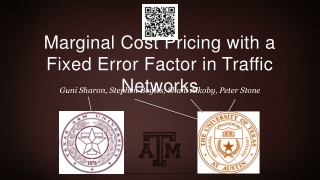DownloadDownload PresentationMarginal Cost Pricing with a Fixed Error Factor in Traffic Networks

# Marginal Cost Pricing with a Fixed Error Factor in Traffic Networks

Télécharger la présentation## Marginal Cost Pricing with a Fixed Error Factor in Traffic Networks

- - - - - - - - - - - - - - - - - - - - - - - - - - - E N D - - - - - - - - - - - - - - - - - - - - - - - - - - -
##### Presentation Transcript

1. Marginal Cost Pricing with a Fixed Error Factor in Traffic Networks Guni Sharon, Stephen Boyles, Shani Alkoby, Peter Stone

2. Our objective 1 Hr 59 Min • Assign routes to drivers such that the total travel time is reduced

3. Background • Previous work showed significant reductions in travel time (up to 46%) when utilizing road pricing • [Mirzaei et al., ITSC-2018; Hanna et al., AAAI-2019; Chen et al., AAAI-2018; Sharon et al., AAMAS-2017] • Why should we care? • In the U.S. alone, congestion cost \$305 billion per year (INRIX) • 1% reduction in traffic congestion = saving \$billions

4. Marginal-cost tolls • Each driver pays his “social cost” (how much his existence hurts others) • When applying Marginal-Cost Toll UE and SO align [Pigou 1920; Beckmann et al. 1956; Braess 1969] 1\$ delay \$1 delay To: turtle \$2 toll

5. Computing MCT • MCT = the marginal delay that each agent will impose on all others • Requires exact knowledge of • Future demand • Roadway capacity conditions • Counterfactual knowledge about traffic conditions without each vehicle • Exact solution is infeasible in real-life

6. Approximating MCT • A recent line of work proposed feasible MCT approximations • [Sharon et al., 2017, Mirzaei et al., 2018, Hanna et al., 2019] • Known: MCT leads to optimal flow • What guarantees can we give for ~MCT ? Promise me that traffic congestion will never worsen

7. ~MCT • - actual tolls • - true MCT • - error factor Assuming uniform error What is the system’s performance (TSTT) as a function of ? 1\$ delay \$1 delay

8. Generalized cost UE (GUE) • A flow feasible for instance is at generalized cost user equilibrium if and only if for every and with , • Drivers follow paths with equal and minimal generalized cost w.r.t. • The GUE can be solved as a convex program…

9. GUE as a convex program S.T. • Proof: the appropriate Lagrangian is: • And the resulting KKT conditions imply GUE: GUE is a linear combination between the SO objective and UE objective (Beckman’s formulation)

10. Attributes of a GUE • Objective: • minimize • Define as the total system travel time at the GUE for error factor = • What is ? • What is ? • What is ?

11. Error in MCT UE SO

12. The paper proves: • THEOREM 1. A GUE flow for an approximate MCT scenario exists and is unique • LEMMA 4. where is a UE solution for a scenario in which the latency affiliated with every path equals • LEMMA 5. For any two errors • LEMMA 6. Any two error values satisfy

13. As a result: UE SO

14. Conclusions: I’m convinced • Underestimating MCT by a constant factor • Traffic will not worsen • Calibrating a parameter that is a multiplier of • E.g., value of time, in Delta-tolling [Sharon et al., 2017] • A local optimum is guaranteed to be a global optimum

15. Appendix

16. Non-uniform error? • Charging inaccurate MCT on link 1 (0.5 true MCT) S T fraction of the flow

17. What can go wrong? • Charging inaccurate MCT on link 1 S T fraction of the flow

18. Existence of GUE flow • If the GUE objective function is strictly convex then there must exist a GUE solution which is unique • Lets examine the Hessian matrix for our objective function: • Since each term evolves only one variable ( is a constant) our Hessian matrix is a diagonal matrix • Each entry along the diagonal corresponds to one link () and equals:

19. Existence of GUE flow • by definition of r • ifis strictly increasing • by definition of flow • ifis twice differentiable and convex

20. Error in MCT • Claim: smaller error () is always better • Theorem: any two error values satisfy and any two error values satisfy • Define where is the GUE flow assuming MCT error • Convince us…

21. I’ll convince you • Define: • Recall that: • We can rewrite the GUE objective function as: • Consider two GUE flows and • minimizes the objective function at • for any feasible and specifically

22. Smaller error is better • if and • In a similar way: • if and • We get: if and and

23. Smaller error is better • We got: if and and • Else if and and : • is continuous and strictly increasing for and for so for any within one of the continuous intervals • So: • for • for